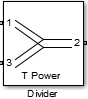# Divider

Model ideal frequency-independent dividers or combiners with S-parameters

• Library:
• RF Blockset / Circuit Envelope / Junctions

•## Description

The Divider block models power dividers or combiners in a Circuit Envelope environment as a S-parameter model. For power dividers, the input power enters at a port, splits, and exits at other ports.

To understand how power dividers are used to model and simulate 66 GHz QPSK RF transmit and receive system with a 32-element hybrid beamforming antenna, see Transmit Subarrays section in Modeling RF mmWave Transmitter with Hybrid Beamforming example.

## Parameters

expand all

Divider type, specified as:

• `T power divider`

The S-parameter matrix for `T-power divider ` is :

$\left[\begin{array}{ccc}{s}_{11}& {s}_{21}& {s}_{31}\\ {s}_{21}& {s}_{22}& {s}_{32}\\ {s}_{31}& {s}_{32}& {s}_{33}\end{array}\right]$

where:

• s11 = (z23 –z1) / (z23 + z1)

• s22 = (z13 –z2) / (z13 + z2)

• s33 = (z12 –z3) / (z12 + z3)

• s21 = (1+ s11) * sqrt(z1 / z2)

• s31 = (1+ s11) * sqrt(z1 / z3)

• s32 = (1+ s22) * sqrt(z2 / z3)

• z12 = z1 * z2 / (z1 + z2)

• z13 = z1 * z3 / (z1 + z3)

• z23 = z2 * z3 / (z2 + z3)

• z1 = Z0(1), z2 = Z0(2), z3 = Z0(3)

• Reference Impedances: Z0 = [z1, z2, z3 ]

• `Resistive power divider`

The S-parameter matrix for `Resistive power divider` is :

$\left[\begin{array}{ccc}0& 1/2& 1/2\\ 1/2& 0& 1/2\\ 1/2& 1/2& 0\end{array}\right]$

When you select this option, the following parameter is available:

• `Wilkinson power divider`

The S-parameter matrix for `Wilkinson power divider` is:

$\left[\begin{array}{ccc}0& -j/\sqrt{2}& -j/\sqrt{2}\\ -j/\sqrt{2}& 0& 0\\ -j/\sqrt{2}& 0& 0\end{array}\right]$

Note

• For DC carrier (0 Hz) frequency, Wilkinson power divider is a zero matrix.

• Starting R2021a, port positions in the Divider block when you select the ```Wilkinson power divider``` component have changed. For more information, see Compatibility Considerations.

• `Tee H-plane (S33=0)`

The `Tee H-plane (S33=0)` is symmetrical, lossless, and reciprocal. The S-parameter matrix is:

$\frac{\left(\begin{array}{ccc}-1& 1& \sqrt{2}\\ 1& -1& \sqrt{2}\\ \sqrt{2}& \sqrt{2}& 0\end{array}\right)}{2}$

• `Tee E-plane`

The `Tee E-plane` is symmetrical, lossless, and reciprocal. The S-parameter matrix is:

$\frac{\left(\begin{array}{ccc}1& 1& \sqrt{2}\\ 1& 1& -\sqrt{2}\\ \sqrt{2}& -\sqrt{2}& 0\end{array}\right)}{2}$

Reference impedance of divider, specified as a positive scalar or three-tuple vector.

Select this parameter to ground and hide the negative terminals. To expose the negative terminals, clear the parameter. By exposing these terminals, you can connect them to other parts of your model.

By default, this option is selected.

## Version History

Introduced in R2014a

expand all

Behavior changed in R2021a